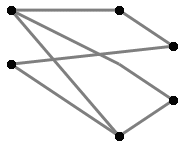### Problem Statement

Graphs are relatively common data structures in computer science. To visualize a graph, we often display it as a bitmap like this one:However, an image is not very easy to work with for most purposes. It is usually much easier to perform common tasks (like finding a shortest path) if the graph is represented as a set of nodes and edges. In this problem, you will be given a graph as a bitmap, and are to convert it to a set of nodes and edges.

You have some information about the bitmap to make this task easier. First, you know that all nodes in the graph are represented by a single pixel of a certain color, which will be represented by an 'N' in the input. Furthermore, each edge will be a single color, represented by an 'E' in the input. The rest of the pixels will be represented by '.'s. After examining the images, you've come up with the following sketch for an algorithm to find and follow edges:
1. First, find an 'N' that is adjacent to an 'E' (either orthogonally, or diagonally).
2. Start following the edge in the same direction as you went to get from the 'N' to the 'E'.
3. Then, as long as the edge has not terminated at an 'N', follow the edge ('E' pixels) by either continuing in the same direction, or if you cannot continue straight, by turning 45 degrees and continuing. You should always go straight when possible, but if you cannot go straight, you will be able to go either 45 degrees left, or 45 degrees right, though not both. In other words, if you cannot go straight, it will be unambiguous which way to turn (this is ensured by the constraints).
4. If, at any point, there is an 'N' straight ahead, or there is not an edge straight ahead and there is an 'N' 45 degrees off from straight ahead, then the edge you are following terminates at that node. Again, this will not be ambiguous, so if there is neither an 'E' nor an 'N' straight ahead, then exactly one of the pixels 45 degrees off from straight will be 'N' or 'E'.
Your task is to write a method parse, which takes a String[], bitmap, each of whose elements represents a row in the bitmap, and returns a String[], each of whose elements represents a single node. To do this, you should first number all of the nodes, starting with the most upper most nodes, and breaking ties by doing the left most first. Your numbering should start at 0. Then, the ith element of the return should be a comma delimited list of all the <edge>s coming out of the ith node. Each <edge> should be formatted as <dest>:<length>, where <dest> is the number of the node to which this edge connects, and <length> is the number of 'E's in the edge. The list of edges in each element should be sorted in ascending order by the index of the element they connect to. Ties should be broken by sorting the edges in ascending order by length.

### Definition

 Class: BitmapToGraph Method: parse Parameters: String[] Returns: String[] Method signature: String[] parse(String[] bitmap) (be sure your method is public)

### Notes

-Only list individual loops (edges from a node to itself) once. See example 4.

### Constraints

-bitmap will contain between 1 and 50 elements, inclusive.
-Each element of bitmap will contain the same number of characters.
-Each element of bitmap will contain between 1 and 50 characters, inclusive.
-Each character of bitmap will be an 'E', an 'N', or a '.'.
-If you follow each edge as described in the problem statement, each edge will terminate at a node.
-There will not be two adjacent 'N's.
-All of the edges will be traversed in the same manner in both directions. In other words, if there is an edge from node i to node j, there will also be an edge from node j to node i which uses all of the same pixels.
-There will be no ambiguity as to which way to go when a 45 turn must be made.

### Examples

0)

 ``` {"NEEE.....N", "....EEEEE.", ".........."}```
`Returns: { "1:8",  "0:8" }`
 The upper left 'N' is node 0, and the upper right 'N' is node 1. There is an edge with 8 'E's connecting them, so element 0 of the return is "1:8" since the edge from 0 to 1 is of length 8. Similarly, element 1 of the return is "0:8"
1)

 ``` {"N.N", ".E.", "N.N"}```
`Returns: { "3:1",  "2:1",  "1:1",  "0:1" }`
 The numbers of the nodes are as follows: ``` 0.1 ... 2.3 ``` Thus, 0 is connected to 3, and 1 is connected to 2.
2)

 ``` {"N..N..N", ".E.E.E.", "..EEE..", "NEEEEEN", "..EEE..", ".E.E.E.", "N..N..N"}```
`Returns: { "7:5",  "6:5",  "5:5",  "4:5",  "3:5",  "2:5",  "1:5",  "0:5" }`
3)

 ``` {".NE....NE..N", "E..E...E.E..", "E..E...E.E.E", ".EE....NE..E"} ```
`Returns: { "0:7",  "3:2,3:4",  "",  "1:2,1:4" }`
 Notice that the loop from node 0 to itself is only listed once. Also note that when there are multiple edges from one node to another, they are sorted by length. Finally, note that there may be edge pixels that are not part of any edge.
4)

 ```{".EE....", "E..E...", "E..E...", "NEEEEE.", "...E..E", "...E..E", "...E..E", "....EE."}```
`Returns: { "0:20" }`
5)

 ```{".EE.", "N..N", ".EE."}```
`Returns: { "1:2,1:2",  "0:2,0:2" }`
6)

 ``` {"N..N.........", ".E.E.........", "..EE....EN...", "...E.N.E.....", "...NEEEEEN...", "...E.N.E.....", "..EE....EN...", ".E.E.........", "N..N........."}```
```Returns:
{ "6:4",
"4:3",
"6:3",
"6:1,7:3,8:4",
"1:3,5:5,9:3",
"4:5",
"0:4,2:3,3:1",
"3:3",
"3:4",
"4:3" }```

#### Problem url:

http://www.topcoder.com/stat?c=problem_statement&pm=1882

#### Problem stats url:

http://www.topcoder.com/tc?module=ProblemDetail&rd=4701&pm=1882

lars2520

#### Testers:

brett1479 , schveiguy

#### Problem categories:

Simulation, String Parsing